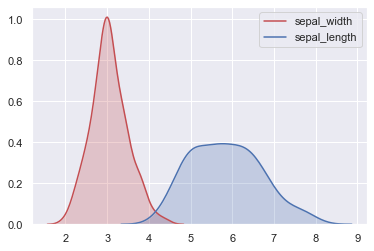# Density plot of several variables

It is sometimes particularly useful to plot the distribution of several variables on the same plot in order to compare them more easily. This can be performed by using the kdeplot function of seaborn several times as presented below.

As stated before, seaborn being built on top of matplotlib enables you to use matplotlib.fig and matplotlib.axes objects to add graphs to your figures.
In the following example, we successively add kdeplots to a matplotlib figure and plot it. From this example, you can play with colors, or any other kdeplot argument to better distinguish the two distributions.

``````# libraries & dataset
import seaborn as sns
import matplotlib.pyplot as plt
# set a grey background (use sns.set_theme() if seaborn version 0.11.0 or above)
sns.set(style="darkgrid")
df = sns.load_dataset('iris')

# plotting both distibutions on the same figure
fig = sns.kdeplot(df['sepal_width'], shade=True, color="r")
fig = sns.kdeplot(df['sepal_length'], shade=True, color="b")
plt.show()``````## Contact & Edit

👋 This document is a work by Yan Holtz. Any feedback is highly encouraged. You can fill an issue on Github, drop me a message onTwitter, or send an email pasting `yan.holtz.data` with `gmail.com`.

This page is just a jupyter notebook, you can edit it here. Please help me making this website better 🙏!